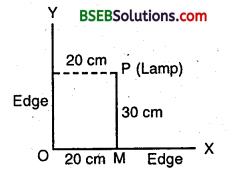# Bihar Board Class 9th Maths Solutions Chapter 3 Coordinate Geometry Ex 3.1

Bihar Board Class 9th Maths Solutions Chapter 3 Coordinate Geometry Ex 3.1 Textbook Questions and Answers.

## BSEB Bihar Board Class 9th Maths Solutions Chapter 3 Coordinate Geometry Ex 3.1Question 1.
How will you describe, the position of a table lamp on your study table to another person?
Solution:
Consider the lamp as a point P and table as a plane. Choose any two perpendicular edges of the table, say OX and OY. Measure the distance of the lamp i.e., P from the longer edge OX, let it be 30 cm. Again, measure the distance of the lamp P from the shorter edge OY, let it be 20 cm.Thus, the position of the lamp P referred to the edges OX and OY is (20, 30).Question 2.
(Street Plan) : A city has two main roads which cross each other at the centre of the’ city. These two roads are along the North-South direction and East- West direction. All-other streets of the city run parallel to these roads and are 200 m apart. There are about 5 streets in each direction. Using 1 cm = 200 m, draw a model of the city on your notebook. Represent the roads/streets by single lines.
There are many cross-streets in your model. A particular cross-street is made by two streets, one running in the North-South direction and another in the East-West direction. Each cross street is referred to in the following manner: If the 2nd street running in the North-South direction and 5th in the East-West direction meet at some crossing, then we will call this cross-street (2, 5). Using this convention, find :
(i) how many cross-streets can be referred to as (4,3)
(ii) how many cross-streets can be referred to as (3, 4)
Solution:
Street plan is as shown in the figure :(i) There is only one cross street, which can be referred as (4, 3).
(ii) There is only one cross street, which can be referred as (3, 4).